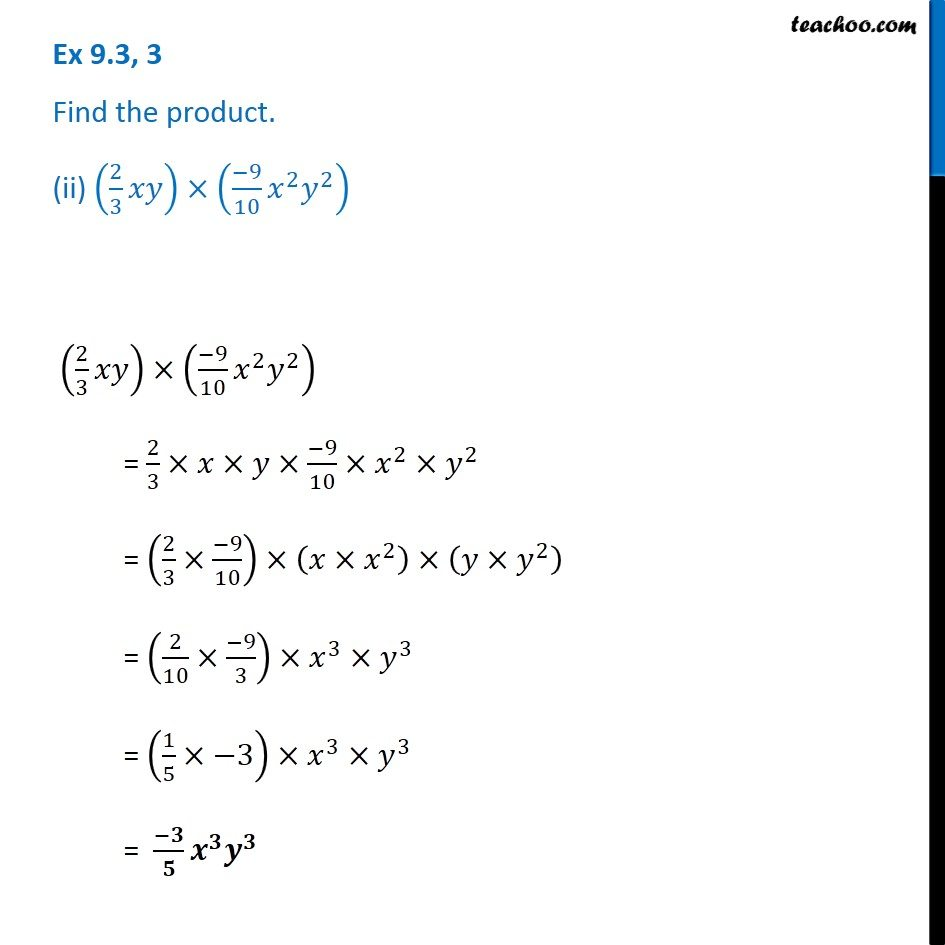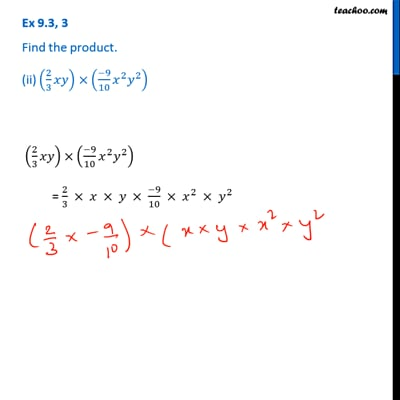Ex 9.3

Chapter 9 Class 8 Algebraic Expressions and Identities
Serial order wiseThis video is only available for Teachoo black users

Get live Maths 1-on-1 Classs - Class 6 to 12

### Transcript

Ex 9.3, 3 Find the product. (ii) (2/3 𝑥𝑦)×((−9)/10 𝑥^2 𝑦^2 ) (2/3 𝑥𝑦)×((−9)/10 𝑥^2 𝑦^2 ) = 2/3×𝑥×𝑦×(−9)/10×𝑥^2×𝑦^2 = (2/3×(−9)/10)×(𝑥×𝑥^2 )×(𝑦×𝑦^2 ) = (2/10×(−9)/3)×𝑥^3×𝑦^3 = (1/5×−3)×𝑥^3×𝑦^3 = (−𝟑)/𝟓 𝒙^𝟑 𝒚^𝟑